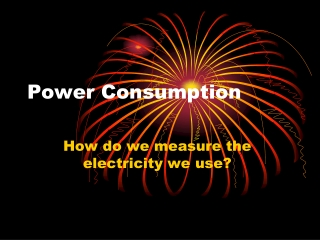DownloadDownload PresentationPower Consumption

# Power Consumption

Télécharger la présentation## Power Consumption

- - - - - - - - - - - - - - - - - - - - - - - - - - - E N D - - - - - - - - - - - - - - - - - - - - - - - - - - -
##### Presentation Transcript

1. Power Consumption How do we measure the electricity we use?

2. What unit do we purchase electricity in? • The electric company bills customers based on the kilo-watt hours of electricity used each month. • Definition • A kilowatt-hour is equal to 1000 watt-hours of electricity.

3. Watt-hours • In order to understand kilo-watt hours, we must first learn what watt-hours are. • Step 1– Determine or calculate the watts used by an appliance. This can be done by reading the nameplate or by using the formula W = V x A.

4. Watt-Hours • Step 2—After determining the watts used, estimate the number of hours the appliance will be used per month. • Step 3—Multiply the watts times the hours of operation to determine watt-hours.

5. Calculations • A 1 horsepower motor uses 1000 watts of electricity for 10 hours. How many watt-hours were consumed by the motor? • 1000 watts x 10 hours = 10,000 watt-hours

6. Calculations • To convert the previous answer to kilowatt hours, simply divide your answer by 1000. • 1 kilowatt-hour is equal to 1000 watt-hours • 10,000 watt-hours / 1000 = 10 kilowatt-hours

7. Perform the following • How many kilowatt-hours would a 100 watt light bulb use in 5 hours?

8. Answer • 100 watt bulb x 5 hours = 500watt-hours • 500 watt-hours/ 1000 = .5 kilowatt-hours

9. Perform the following: • How many kilowatt-hours of electricity would a 120 watt mixer use in 15 minutes of operation?

10. Answer • 15 minutes = ¼ hour • 120 watts x ¼ hour = 30 watt-hours • 30 watt-hours/1000 = .030 kilowatt-hours

11. Summary • Although we may calculate watt-hours for a piece of equipment or appliance, these are such small units that we must convert them into the kilowatt-hour unit used by the power company in our particular area.